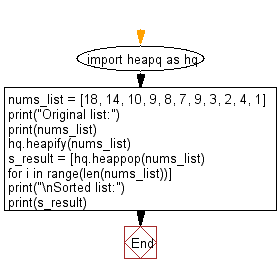﻿ Python heap queue algorithm: Sort a given list of elements in ascending order using Heap queue algorithm - w3resource

# Python: Sort a given list of elements in ascending order using Heap queue algorithm

## Python heap queue algorithm: Exercise-6 with Solution

Write a Python program to sort a given list of elements in ascending order using the heap queue algorithm.

Sample Solution:

Python Code:

``````import heapq as hq
nums_list = [18, 14, 10, 9, 8, 7, 9, 3, 2, 4, 1]
print("Original list:")
print(nums_list)
hq.heapify(nums_list)
s_result = [hq.heappop(nums_list) for i in range(len(nums_list))]
print("\nSorted list:")
print(s_result)
```
```

Sample Output:

```Original list:
[18, 14, 10, 9, 8, 7, 9, 3, 2, 4, 1]

Sorted list:
[1, 2, 3, 4, 7, 8, 9, 9, 10, 14, 18]
```

Flowchart:Python Code Editor:

Have another way to solve this solution? Contribute your code (and comments) through Disqus.

What is the difficulty level of this exercise?

Test your Programming skills with w3resource's quiz.

﻿